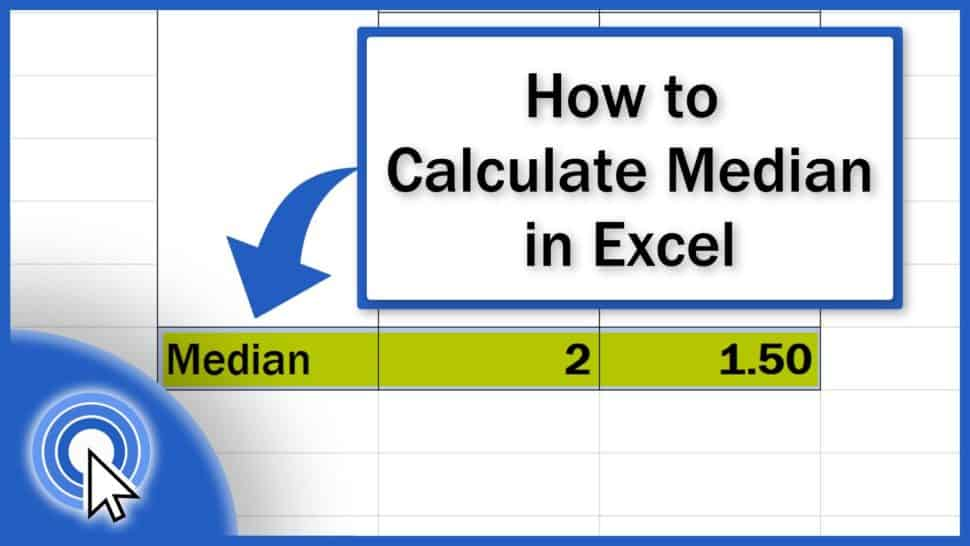# How to Calculate the Median in Excel (Quick and Easy)In today’s tutorial, we’re going to go through how to calculate the median in Excel. You’ll see a quick and easy way to find out the midpoint in any set of numbers.

Let’s start!

Would you rather watch this tutorial? Click the play button below!

Contents

## How to Calculate the Median in Excel

To see how to calculate the median, we’ll be working with these two sets of numbers – A and B. Each of the two examples will guide you through some interesting tricks that are good to know when calculating the median.

So, let’s start with the example A. The set consists of an odd number of items, so we’ll be calculating the median of the values 1, 2 and 3.

To insert the right formula, start typing the equal sign and ‘MEDIAN’ right after. As usual, Excel will look the function up. Click on it and simply enter the set of numbers for the calculation of the median – in this case, the column A.

Let’s close the brackets, press Enter and here we go!

Quick and easy – Excel can find the median in any set of numbers immediately. You don’t have to manually sort the numbers first and then look for the median value located at the midpoint of the sorted list, which is number 2 in this case. Isn’t that great?

## Calculating the Median with Blank Data Cells

It’s also good to know that Excel can calculate the median even though the list of numbers contains blank data. So, if there are any blank cells in the column containing the set, it’s all okay to run the formula and you won’t have to worry about these.

## How to Find Out the Median with Accuracy

In the second example, we’ll have a look at how to find out the median with accuracy.

Let’s calculate the median the same way we did a while ago – enter the equal sign, add the reference to the relevant set of numbers, close the brackets, hit Enter and here’s the result.

But the set of numbers we’re working with right now contains an even number of items, which means the midpoint is between two values. In this case, it’s quite handy to format the cell to show decimal places. That way you’ll see the accurate value of median, not the rounded result.

As you can see here, the median is not 2, but 1.5 and was calculated as the value between the two numbers located in the middle, which are 1 and 2. That’s why the median from the number set 0, 1, 2, and 3 is 1.5.

It’s important to note that Excel treats 0 like a regular value, so it’s taken into account in the calculation.

Don’t miss out a great opportunity to learn:

If you found this tutorial helpful, give us a like and watch other tutorials by EasyClick Academy. Learn how to use Excel in a quick and easy way!

Is this your first time on EasyClick? We’ll be more than happy to welcome you in our online community. Hit that Subscribe button and join the EasyClickers!

Thanks for watching and I’ll see you in the next tutorial!